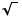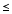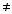A program for the TI-82/83 that factors integers.

Ken Levasseur
UMass Lowell
Kenneth_Levasseur@uml.edu

Given an integer N, this program facors N with format {p1,e1,p2,e2,...} where

`N = p1^e1  p2^e2 ...`

This program is required by a related program to compute Euler's Phi function. The EulerP program uses the variable O ("Oh") that appears in the third line of this program.

Program:Factor
``` 0->dim(L1)
Prompt N
N->O
1->S
2->F
0->E(N)->M
While FM
While fPart((N/F))=0
E+1->E
N/F->N
End
If E>0:Then
F->L1(S)
E->L1(S+1)
S+2->S
0->E(N)->M
End
If F=2
Then:3->F
Else:F+2->F
End
End
If N1:Then
N->L1(S)
1->L1(S+1)
End
L1```

A program for the TI-82/83 that Euler's Phi Function.

The euler function, phi, is defined by phi(n) = the number of integers in the set {1, 2, ..., n-1} that are relatively prime to n. The program is quite short, but uses another program, factor

```Program: EulerP
:prgmFactor
:O*prod((1-(1/seq(L1(K),K,1,Dim(L1)-1,2)))
```

Back to the UML TI program page